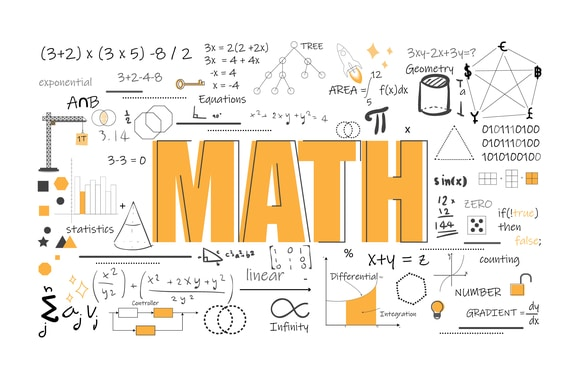# Numbers In Base Two

Welcome to Class !!

In today’s Mathematics class, We will be discussing Numbers in Base 2. We hope you enjoy the class!BINARY NUMBERS (BASE 2 NUMBERS)

• Subtraction in base 2
• Multiplication & Division in base 2

We can add binary numbers in the same way as we separate with ordinary base 10 numbers.

The identities to remember are:-

0 + 0 = 0, 0 + 1 = 1, 1 + 0 = 1, 1 + 1 = 10, 1 + 1 + 1 = 11, 1 + 1 + 1 + 1 = 100

Worked Examples

Simplify the following

1. 1110 + 1001 2.         1111 + 1101 + 101

Solutions:

Note: 11 take 1 carry 1

10 take 0 carry 1

100 take 0 carry 10

EVALUATION

1. Simplify the following 101 + 101 +111
2. 10101 + 111

In bicimals, the binary point is placed underneath each other exactly the same way like ordinary decimals.

Example:

1. 1.1011two +   10.1001two  +  10.01
2. 10.001two+ 101.111

Solution:

]

#### SUBTRACTION IN BASE TWO

The identities to remember on subtraction are: 0 – 0 = 0, 1 – 0 = 1, 10 – 1 = 1, 11 – 1 = 10, 100 – 1 = 11

Worked Examples

Simplify the following:-

(a)      1110 – 1001

Solutions:

SUBTRACTION IN BICIMAL

Example

101.101two – 11.011two

$solution:\phantom{\rule{0ex}{0ex}}\phantom{\rule{0ex}{0ex}}101.101\phantom{\rule{0ex}{0ex}}\frac{11.011}{{\frac{10.010}{}}_{}}$

EVALUATION

1. 10111÷110
2. 10001 x 11

We have come to the end of this class. We do hope you enjoyed the class?

Should you have any further question, feel free to ask in the comment section below and trust us to respond as soon as possible.

In our next class, we will be talking about Numbers in Base II. We are very much eager to meet you there.

Are you a Parent? Share your quick opinion and win free 2-month Premium Subscription

### 3 thoughts on “Numbers In Base Two”

1.I don’t get it

2.Bello Abdulazeez omotayo

Useful to learn

1.There is no plenty assignment

Don`t copy text!